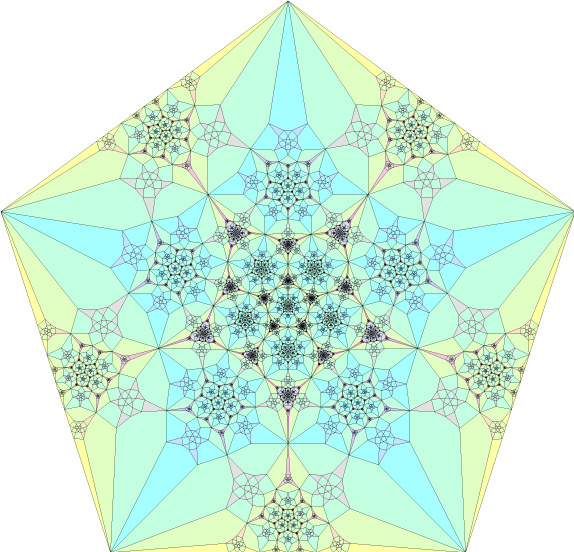# SymmetrySymmetry is a concept in mathematics that refers to the invariance of an object under certain transformations. It is an important concept in many areas of mathematics, as well as in physics, engineering, and art. Symmetry can be thought of as a balance or repetition of features in a design, pattern, or object.
In mathematics, symmetry can be described as a transformation that leaves an object unchanged. For example, rotating a square by 90 degrees results in an image that is indistinguishable from the original square. This property is known as rotational symmetry. Other types of symmetry include reflection symmetry, translation symmetry, and scaling symmetry.
One of the most important contributions to the study of symmetry was made by the German mathematician Emmy Noether. In 1915, she showed that the principles of energy conservation could be seen as an underlying symmetry in physical systems. Noether’s theorem states that every continuous symmetry of a physical system corresponds to a conservation law. For example, the conservation of energy is a result of the time-translational symmetry of a physical system. This connection between symmetry and conservation laws has been applied in a variety of fields, including quantum mechanics, particle physics, and cosmology.
The mathematics of symmetry also plays a crucial role in the study of crystallography. Crystals are materials that have a repeating pattern of atoms, ions, or molecules. These patterns can be described mathematically as symmetrical arrangements of atoms in space. Understanding the symmetries of crystals is important for understanding their properties, such as their electrical conductivity and mechanical strength.
Symmetry is also used in the study of group theory, a branch of mathematics that studies symmetry groups. In group theory, a group is a set of elements that can be combined in a particular way to form a new element that is also in the set. For example, the set of rotations of a square forms a group, as rotations can be combined to form new rotations.
In art and design, symmetry is often used to create aesthetically pleasing and balanced compositions. For example, in architecture, symmetrical designs are used to create buildings with a sense of order and harmony. In visual arts, symmetry is used in painting, drawing, and other forms of art to create images that are balanced and aesthetically pleasing.
In conclusion, symmetry is a concept in mathematics that refers to the invariance of an object under certain transformations. It is a fundamental concept in many areas of mathematics, physics, engineering, and art. Emmy Noether’s discovery of the connection between symmetry and conservation laws has had a profound impact on the study of physical systems. The mathematics of symmetry is used in the study of crystallography, group theory, and art and design, and it plays a crucial role in understanding the properties of objects and systems.